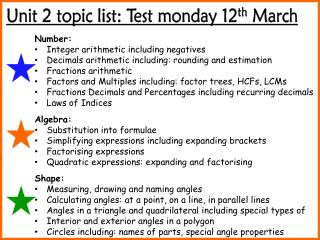DownloadDownload PresentationNumber: Integer arithmetic including negatives

# Number: Integer arithmetic including negatives

Download Presentation## Number: Integer arithmetic including negatives

- - - - - - - - - - - - - - - - - - - - - - - - - - - E N D - - - - - - - - - - - - - - - - - - - - - - - - - - -
##### Presentation Transcript

1. Unit 2 topic list: Test monday 12th March • Number: • Integer arithmetic including negatives • Decimals arithmetic including: rounding and estimation • Fractions arithmetic • Factors and Multiples including: factor trees, HCFs, LCMs • Fractions Decimals and Percentages including recurring decimals • Laws of Indices • Algebra: • Substitution into formulae • Simplifying expressions including expanding brackets • Factorising expressions • Quadratic expressions: expanding and factorising • Shape: • Measuring, drawing and naming angles • Calculating angles: at a point, on a line, in parallel lines • Angles in a triangle and quadrilateral including special types of • Interior and exterior angles in a polygon • Circles including: names of parts, special angle properties